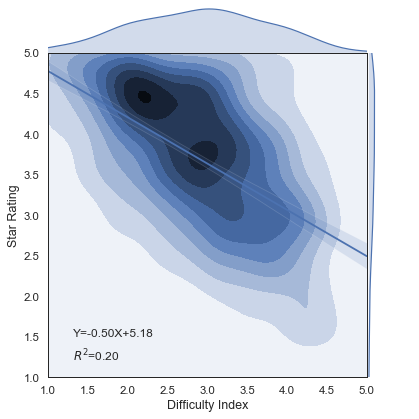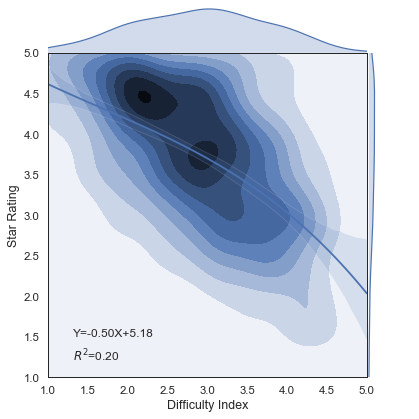seaborn画图为什么回归线不拟合散点分布？``````    df = pd.read_csv(src,nrows=10000, usecols=['professor_name','school_name', 'star_rating', 'diff_index'])
pd.set_option('display.max_rows', 100, 'display.max_columns', 1000, "display.max_colwidth", 1000, 'display.width',
1000)
df = df[(df['star_rating'] >= 1.0 ) & (df['diff_index'] >= 1.0)].drop_duplicates(['professor_name','school_name'], 'first', False)
print(df.describe()) #计算平均数，标准差

# 计算评分和难度的回归方程
print('计算diff_index和star_rating的回归方程和R方')
data = df[['diff_index', 'star_rating']]
regression = stats.linregress(data)
print("R square：", regression ** 2)
print('线性回归方程是 Y= %.3fX + %.3f,rvalue是%.3f,p-values是%s,标准误是%s' % regression)
g = sns.set("paper",font_scale =1.3)
g = sns.set_style("white")

data = data.rename(index=str, columns={'diff_index': 'Difficulty Index', 'star_rating': 'Star Rating'})

g = sns.jointplot('Difficulty Index','Star Rating', data=data,height=6,ratio=7, kind="kde", xlim=(1,5), ylim=(1.0, 5.0),space=0,palette=sns.color_palette('Blues'))
g = sns.regplot(data['Difficulty Index'], data['Star Rating'], scatter=False, ax=g.ax_joint)

plt.text(-20.8, 1.5, r"Y=-0.50X+5.18", fontsize=12)
plt.text(-20.8, 1.2, r'\$R^2\$=0.20', fontsize=12)
plt.show()
``````